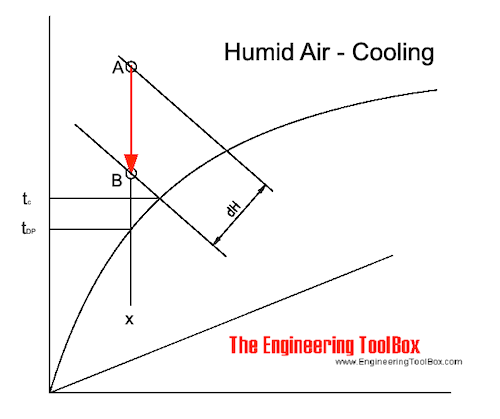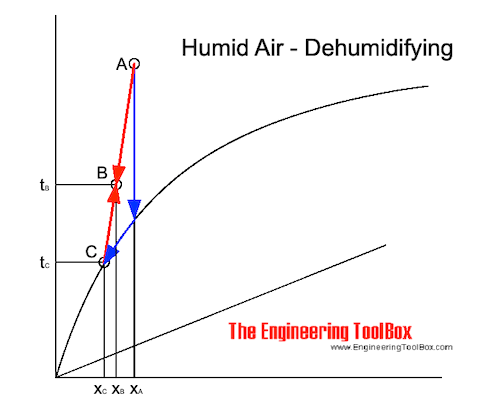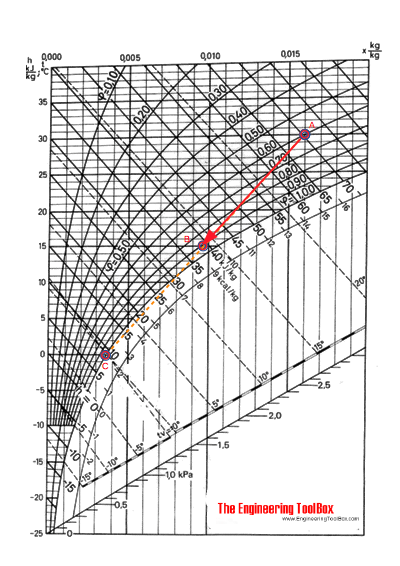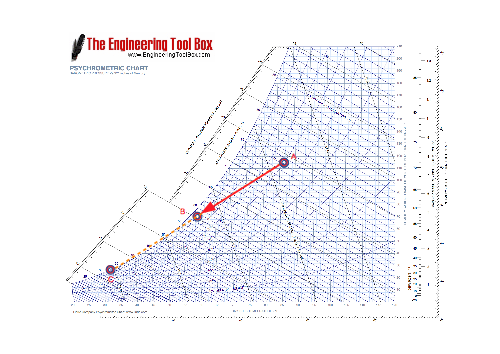Engineering ToolBox - Resources, Tools and Basic Information for Engineering and Design of Technical Applications!

# Moist Air - Cooling and Dehumidifying

## Cooling and dehumidifying processes of moist and humid air - sensible and latent cooling.

When cooling air - part of the energy is used to separate the water (latent heat), and the rest is used to lower the air temperature (sensible heat).

### Cooling Moist Air - Sensible Cooling

If the temperature on a cooling surface - tC - is above or equal to the dew point temperature - tDP - of the surrounding air, the air will be cooled without any change in specific humidity. It is Sensible Heat - the "temperature heat" - in the air that is removed.

The air cools along a constant specific humidity - x - line as indicated in the Mollier diagram below:The process is very similar (opposite direction) to the sensible heating process and the heating formulas can be adapted to calculate change in enthalpy and temperature.

Note! The specific humidity is constant but the relative humidity will increase.

### Dehumidifying Moist Air - Latent Cooling

If the temperature on a cold surface is lower than the dew point temperature - tDP - of the humid air, vapor in the air condensates on the surface. Latent heat - vapor - is removed from the humid air.

This process is indicated in the Mollier diagram as below. The air cools in the direction of point C, which is the intersection point of the cold surface temperature (the cooling surface dew point temperature or the apparatus dew-point - tADP) and the saturation line.With a cold surface of unlimited size and a very small amount of air, it would be possible to reach point C. In the real world a limited surface is never 100% effective and the final state of the cooled and dehumidified air will be somewhere on the straight line between point A and C - point B.

The amount of condensated vapor will be the difference in specific humidity xA - xB.

Note! This process decreases the specific humidity and increases the relative humidity.

### Contact Factor - β

The efficiency of a cooling coil can be expressed with the Contact Factor - β - as

β = (xA - xB) / (xA - xC)

= (hA - hB) / (hA - hC)

≈ (tA - tB) / (tA - tC)                      (1)

where

β = Contact Factor

x = specific humidity (kg/kg)

h = enthalpy (kJ/kg)

t = temperature (oC)

### Bypass Factor - BPF

The Bypass Factor - BPF - (or BF) is also used to express cooling coil efficiency as

BPF = (hB - hC) / (hA - hC)

= (tB - tC) / (tA - tC)

= (xB - xC) / (xA - xC)                       (2)

where

BPF = Bypass Factor (BF)

The relationship between the Contact Factor and the Bypass Factor can be expressed as

BPF = 1 - β         (3)

### Heat Flow in a Cooling Coil

The total heat flow rate through a cooling coil can be calculated as

q = m (hA - hB)                    (4)

where

q = heat flow rate (kJ/s, kW)

m = mass flow rate of air (kg/s)

The total heat flow can be expressed as

qs = v ρ (hA - hB)                        (4a)

where

v = volume flow (m3/s)

ρ = density of air (kg/m3)

Note! The density of air varies with temperature. At 0oC and atmospheric pressure the density is 1.293 kg/m3. At 80oC the density is 1.0 kg/m3.

The total heat flow rate can be split into sensible and latent heat. The sensible heat flow rate can be expressed as

qs = m cp (tA - tB)                      (4b)

where

cp = 1.01 - specific heat air (kJ/kgoC)

The latent heat flow rate can be expressed as

qs = m hwe (xA - xB)                    (4c)

where

hwe = water evaporation enthalpy (2502 kJ/kg)

### Example - Cooling and Dehumidifying Air

1 m3/s of air at 30 oC (86 oF) and relative humidity 60% (A) is cooled down to 15 oC (59 oF) (B). The surface temperature of the cooling coil is 0 oC (32 oF) (C). The density of the air at 20 oC is 1.205 kg/m3.

In the Mollier diagram the state of the cooled air (B) is in the intersection between the straight line between (A) and (C) and the 15 oC temperature line.From the Mollier diagram it can be stated that the enthalpy in (A) is 70 kJ/kg, in (B) 38.5 kJ/kg and in (C) 8.5 kJ/kg.

The Contact Factor can be calculated as

β = ((70 kJ/kg) - (38.5 kJ/kg)) / ((70 kJ/kg) - (8.5 kJ/kg))

= 0.51

The total heat flow can be calculated as

q = (1 m3/s) (1.205 kg/m3) ((70 kJ/kg) - (38.5 kJ/kg))

= 38 (kJ/s, kW)

The sensible heat flow can be calculated as

qs = (1 m3/s) (1.205 kg/m3) (1.01 kJ/kg.oC) (30oC - 15oC)

= 18.3 (kW)

According the Mollier diagram the specific humidity in (A) is 0.016 kg/kg and in (B) 0.0096 kg/kg and the latent heat flow can be calculated as

qs = (1 m3/s) (1.205 kg/m3) (2502 kJ/kg) ((0.016 kg/kg) - (0.0096 kg/kg))

= 19.3 (kW)

Note! Due to inaccuracies when working with graphical diagrams there is a small difference between the total heat flow and the sum of the latent and sensible heat. Inaccuracies like this are in general within acceptable limits.

A similar cooling and dehumidifying process in the psychrometric chart:## Related Topics

• ### Air Psychrometrics

Moist and humid air - psychrometric charts, Mollier diagrams, air-condition temperatures and absolute and relative humidity and moisture content.

## Related Documents

• ### Air - Absolute Humidity

Absolute humidity is the actual mass of water vapor present in the air water vapor mixture.
• ### Air - Heating, Cooling, Mixing, Humidifying or Dehumidifying Processes

Basic air changing state processes - heating, cooling, mixing, humidifying and dehumidifying by adding steam or water - psychometric diagrams and the Mollier charts.
• ### Air - Humidifying by Adding Steam or Water

Air can be humidified by adding water or steam.
• ### Air - Humidifying with Steam, SI units

Using steam to humidify air.
• ### Air - Humidity Ratio

The mass of water vapor present in moist air - to the mass of dry air.
• ### Air - Maximum Moisture Carrying Capacity

Maximum water content in humid air vs. temperature.
• ### Air - Moisture Holding Capacity vs. Temperature

The moisture holding capacity of air increases with temperature.
• ### Air Conditioning - Cooling of Air and Condensate Generated

Water may condensate when air is cooled in air conditioning systems.
• ### Air Cooling of Storage Roooms

Heat removed from storage rooms with cooled air.
• ### Cooling Tower Efficiency

Maximum cooling tower efficiency is limited by the cooling air wet-bulb temperature.
• ### Dehumidifiers

Classification of dehumidifiers.
• ### Dry Bulb, Wet Bulb and Dew Point Temperatures

Dry Bulb, Wet Bulb and Dew Point temperatures can be used to determine the state of humid air.
• ### Great Sensible Heat Factor - GSHF

The Great Sensible Heat Factor is the ratio sensible to total heat in a cooling coil.
• ### Heat Recovery Efficiency

Classification of heat recovery efficiencies - temperature efficiency, moisture efficiency and enthalpy efficiency - online heat exchanger efficiency calculator.
• ### Humid Air - Heating

Enthalpy change and temperature rise when heating humid air without adding moisture.
• ### Moist Air - Psychrometric Table for Pressure 29.92 inHg

Dry and wet bulb temperatures, saturation pressure, water vapor weight, specific volume, heat and more.
• ### Moist Air - Relative Humidity

Relative humidity in moist air is the ratio of partial vapor pressure to air pressure.
• ### Moist Air - the Mollier Diagram

The Mollier diagram is a graphic representation of the relationship between air temperature, moisture content and enthalpy - and is a basic design tool for building engineers and designers.
• ### Moist Air - Transforming the Mollier Diagram to a Psychrometric Chart - or vice versa

The relationship between the psycrometric chart and the Mollier diagram.

## Engineering ToolBox - SketchUp Extension - Online 3D modeling!

Add standard and customized parametric components - like flange beams, lumbers, piping, stairs and more - to your Sketchup model with the Engineering ToolBox - SketchUp Extension - enabled for use with older versions of the amazing SketchUp Make and the newer "up to date" SketchUp Pro . Add the Engineering ToolBox extension to your SketchUp Make/Pro from the Extension Warehouse !

We don't collect information from our users. More about

## Citation

• The Engineering ToolBox (2004). Moist Air - Cooling and Dehumidifying. [online] Available at: https://www.engineeringtoolbox.com/cooling-dehumidifying-air-d_695.html [Accessed Day Month Year].

Modify the access date according your visit.

9.27.11